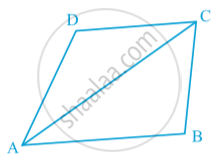Share

# Angle Sum Property of a Quadrilateral

#### notes

The sum of the angles of a quadrilateral is 360º. This can be verified by drawing a diagonal and dividing the quadrilateral into two triangles.  Let ABCD be a quadrilateral and AC be a diagonal in following fig.You know that
∠ DAC + ∠ ACD + ∠ D = 180° (1)
Similarly, in ∆ ABC,
∠ CAB + ∠ ACB + ∠ B = 180° (2)
Adding (1) and (2), we get
∠ DAC + ∠ ACD + ∠ D + ∠ CAB + ∠ ACB + ∠ B = 180° + 180° = 360°
Also, ∠ DAC + ∠ CAB = ∠ A and ∠ ACD + ∠ ACB  = ∠ C
So, ∠ A + ∠ D + ∠ B + ∠ C = 360°.
i.e., the sum of the angles of a quadrilateral is 360°.

### Shaalaa.com

Angle Sum Property of Quadrilaterals [00:04:53]
S
0%

S